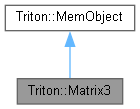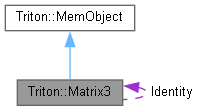Triton Visual simulation library for ocean rendering.
Triton::Matrix3 Class Reference

A simple 3x3 matrix class and its operations. More...

#include <Matrix3.h>

Inheritance diagram for Triton::Matrix3:[legend]
Collaboration diagram for Triton::Matrix3:[legend]

Public Member Functions

Matrix3 ()
Default contructor; performs no initialization for efficiency. More...

Matrix3 (double e11, double e12, double e13, double e21, double e22, double e23, double e31, double e32, double e33)
Constructor that instantiates the 3x3 matrix with initial values. More...

Matrix3 (double *m)
Constructor that takes an array of 9 doubles in row-major order. More...

~Matrix3 ()
Destructor. More...

void TRITONAPI ToFloatArray (float val) const
Returns a static 3x3 float array in row major order. More...

Populates the matrix to model a rotation about the X axis by a given amount, in radians. More...

Populates the matrix to model a rotation about the Y axis by a given amount, in radians. More...

Populates the matrix to model a rotation about the Z axis by a give amount, in radians. More...

void TRITONAPI FromXYZ (double Rx, double Ry, double Rz)
Populates the matrix as a series of rotations about the X, Y, and Z axes (in that order) by specified amounts in radians. More...

Matrix3 TRITONAPI operator * (const Matrix3 &mat)
Multiplies two matrices together. More...

Vector3 TRITONAPI operator * (const Vector3 &rkVector) const
Multiplies the matrix by a vector, yielding another 3x1 vector. More...

Matrix3 TRITONAPI Transpose () const
Caculate the inverse of the matrix. More...

Public Attributes

double elem 
The data members are public for convenience.

Friends

Vector3 TRITONAPI operator * (const Vector3 &vec, const Matrix3 &mat)
Multiplies a 1x3 vector by a matrix, yielding a 1x3 vector. More...

Detailed Description

A simple 3x3 matrix class and its operations.

◆ Matrix3() [1/3]

 Triton::Matrix3::Matrix3 ( )
inline

Default contructor; performs no initialization for efficiency.

◆ Matrix3() [2/3]

 Triton::Matrix3::Matrix3 ( double e11, double e12, double e13, double e21, double e22, double e23, double e31, double e32, double e33 )
inline

Constructor that instantiates the 3x3 matrix with initial values.

◆ Matrix3() [3/3]

 Triton::Matrix3::Matrix3 ( double * m )
inline

Constructor that takes an array of 9 doubles in row-major order.

◆ ~Matrix3()

 Triton::Matrix3::~Matrix3 ( )
inline

Destructor.

◆ FromRx()

 void TRITONAPI Triton::Matrix3::FromRx ( double rad )

Populates the matrix to model a rotation about the X axis by a given amount, in radians.

◆ FromRy()

 void TRITONAPI Triton::Matrix3::FromRy ( double rad )

Populates the matrix to model a rotation about the Y axis by a given amount, in radians.

◆ FromRz()

 void TRITONAPI Triton::Matrix3::FromRz ( double rad )

Populates the matrix to model a rotation about the Z axis by a give amount, in radians.

◆ FromXYZ()

 void TRITONAPI Triton::Matrix3::FromXYZ ( double Rx, double Ry, double Rz )

Populates the matrix as a series of rotations about the X, Y, and Z axes (in that order) by specified amounts in radians.

◆ operator *() [1/2]

 Matrix3 TRITONAPI Triton::Matrix3::operator * ( const Matrix3 & mat )

Multiplies two matrices together.

◆ operator *() [2/2]

 Vector3 TRITONAPI Triton::Matrix3::operator * ( const Vector3 & rkVector ) const

Multiplies the matrix by a vector, yielding another 3x1 vector.

◆ ToFloatArray()

 void TRITONAPI Triton::Matrix3::ToFloatArray ( float val ) const
inline

Returns a static 3x3 float array in row major order.

◆ Transpose()

 Matrix3 TRITONAPI Triton::Matrix3::Transpose ( ) const

Caculate the inverse of the matrix.

◆ operator *

 Vector3 TRITONAPI operator * ( const Vector3 & vec, const Matrix3 & mat )
friend

Multiplies a 1x3 vector by a matrix, yielding a 1x3 vector.

The documentation for this class was generated from the following file: